# JEE Main Mathematics Mock - 5

## 25 Questions MCQ Test Mock Test Series for JEE Main & Advanced 2022 | JEE Main Mathematics Mock - 5

Description
Attempt JEE Main Mathematics Mock - 5 | 25 questions in 60 minutes | Mock test for JEE preparation | Free important questions MCQ to study Mock Test Series for JEE Main & Advanced 2022 for JEE Exam | Download free PDF with solutions
QUESTION: 1

Solution:
QUESTION: 2

Solution:
QUESTION: 3

### [(1+cosθ+i sinθ)/(i+sinθ+i cosθ)]4 = cos nθ + isin nθ, then n=

Solution:
QUESTION: 4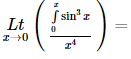Solution:
QUESTION: 5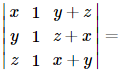Solution:
QUESTION: 6

The differential equation which represents the family of plane curves y=exp. (cx) is

Solution: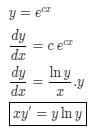QUESTION: 7

If the domain of function f(x) = x2 - 6x + 7 is (-∞, ∞), then the range of function is :

Solution:
QUESTION: 8

In the following question, a Statement-1 is given followed by a corresponding Statement-2 just below it. Read the statements carefully and mark the correct answer-
Let a, b, c, p, q be real numbers. Suppose α,β are the roots of the equation x2 + 2px + q = 0 and a, 1/β are the roots of the equation ax2 + 2bx + c = 0, where β2 ∉ {-1, 0, 1}
Statement-1:
(p2-q)(b2-ac)≥0
Statement-2:
b≠pa or c≠qa

Solution: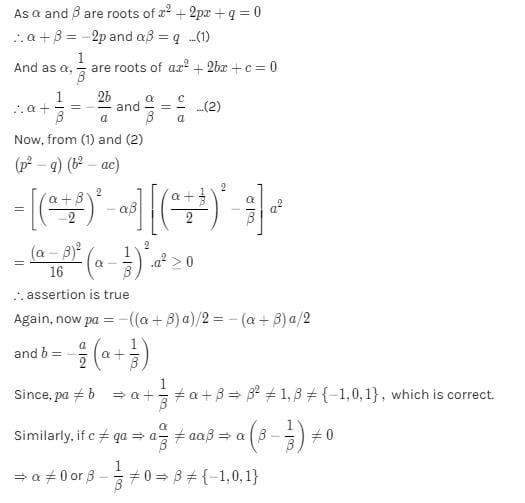QUESTION: 9

The angle between the pair of tangents drawn from the point (1,2) to the ellipse 3x2 + 2y2= 5 is

Solution:
QUESTION: 10

(d/dx)[cos(1-x2)2]=

Solution:
QUESTION: 11

In the following question, a Statement of Assertion (A) is given followed by a corresponding Reason (R) just below it. Read the Statements carefully and mark the correct answer-
Assertion(A): ex , loge x are two functions such that each is the image of the other with respect to the line x = y
Reason (R): The inverse of every bijective function is symmetric about the line x = y

Solution:
QUESTION: 12

A square tank of capacity 250 cubic m has to be dug out. The cost of land is Rs 50 per sq.m. The cost of digging increases with the depth and for the whole tank is 400 (depth)2 rupees. The dimensions of the tank for the least total cost are

Solution:
QUESTION: 13

The same roots of 3-4 i are

Solution:
QUESTION: 14

Everybody in a room shakes hands with every body else. The total number of hand shakes is 66. Then the number of persons in the room is

Solution:

There are n people in the room
n(n - 1)/2 = 66
n2 - n = 132
n2 - n - 132 = 0
n2 - 12n + 11n - 132 = 0
n(n - 12) + 11(n - 12) = 0
(n + 11) (n - 12) = 0
n = 12, - 11
Hence total no of persons = 12

QUESTION: 15

If the sum of the squares of the roots of x2 + px - 3 = 0 is 10, then the values of p =

Solution: Sum of the roots of equation :- α + β = - b/aProducts of the roots of equation :- αβ = c/a squaring on both side sum of roots of equation α^2 +β^2 +2αβ = b^2/a^2 put values in above equation 10 + 2(-3) =b^2 b = +2 or b = -2
QUESTION: 16

Three identical dice are rolled. The probability that the same number will appear on each of them, is

Solution:
QUESTION: 17

If A is the single A.M. between two numbers a and b and S is the sum of n A.M.'s between them, then S/A depends upon

Solution:
QUESTION: 18
If the two lines of regression are 5x + 3y = 55 and 7x + y = 45, then the correlation coefficient between x and y is
Solution:
QUESTION: 19

M telegrams are distributed at random over N communication channels (N > M). The probability of the event
A = {not more than one telegram will be sent over any channel} is

Solution:

The total no. of ways of distributing M telegrams over N channels = NM
The number of ways of choosing M channels out of N to send one telegram over each channel = NCM
∴ Total no. of ways to send M telegrams over each channel = NCM . M!
∴ Required probubility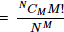QUESTION: 20

The equation of a line passing through (-a,0) and form a triangle of area 'T' with coordinates axes, is

Solution:
*Answer can only contain numeric values
QUESTION: 21

If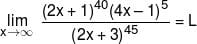then value of L/128 is :-

Solution: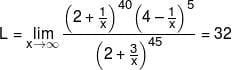*Answer can only contain numeric values
QUESTION: 22

If the function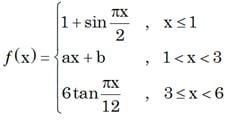is continuous in the interval (–∞, 6) then value of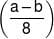is

Solution: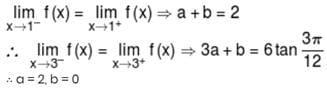*Answer can only contain numeric values
QUESTION: 23

Value of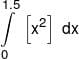is equals to
(where [.] denotes greatest integer function)

Solution: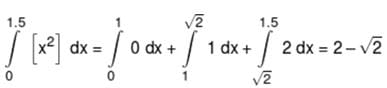*Answer can only contain numeric values
QUESTION: 24

If the area bounded by curve x + |y| = 1 and the y-axis is k, then k/4 is equals to

Solution: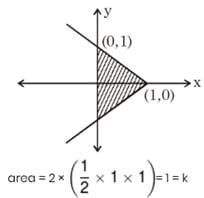*Answer can only contain numeric values
QUESTION: 25

If value of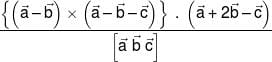is k then k/4 is

Solution: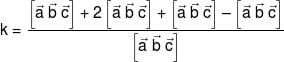k = 3Use Code STAYHOME200 and get INR 200 additional OFF Use Coupon Code